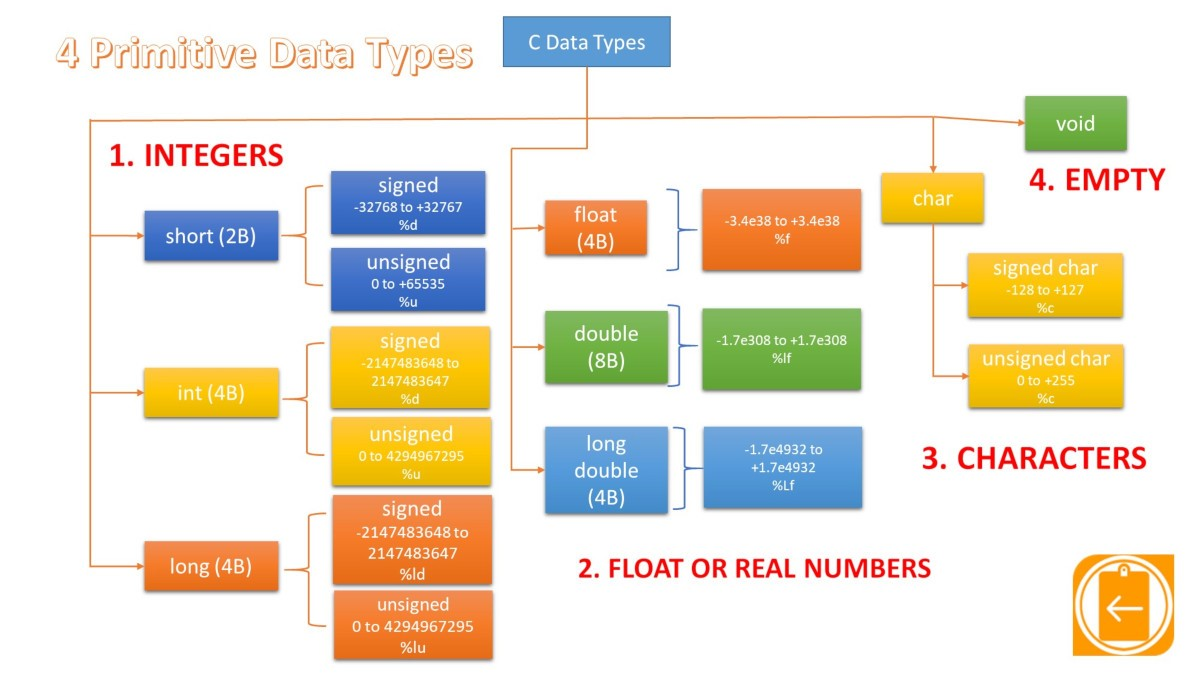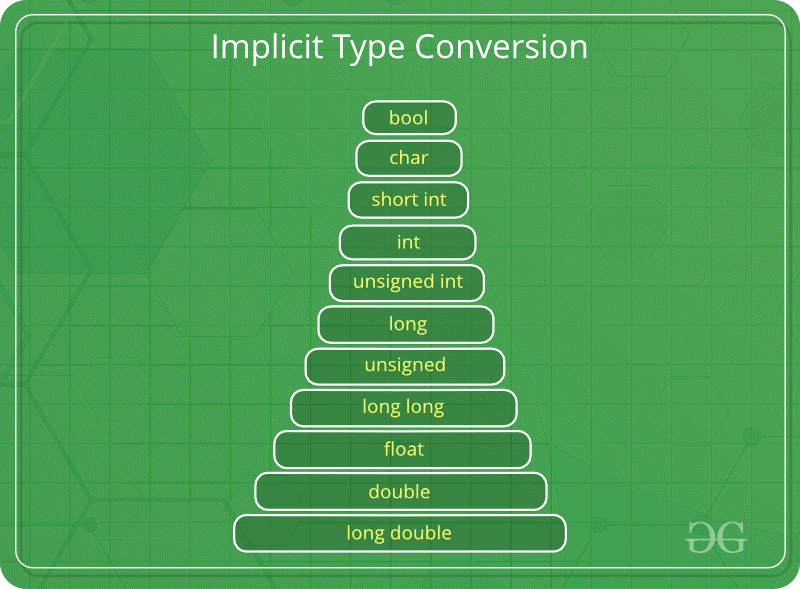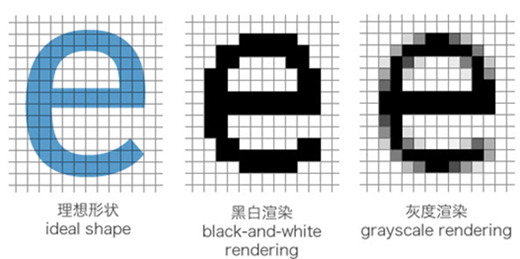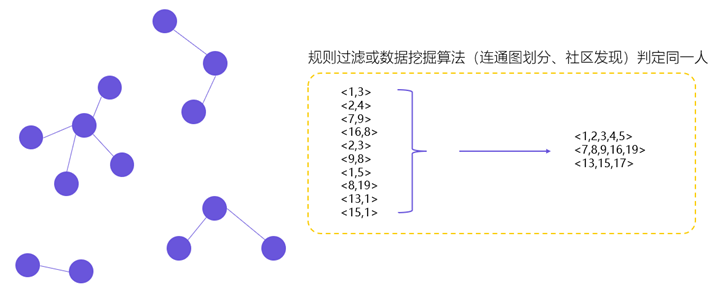C语言：基本数据类型基本数据类型

整型 int

C语言的整型表示的是整数，分为有符号（int）和无符号（unsigned int）。其中无符号整型只能表示非负整数（自然数），不管是有符号还是没符号都存在极限值（表示的数字不能超过或小于某个值），而在C语言中，极限值并不是固定的，不同硬件、操作系统、编译器都可能有不同的值。想要了解当前C语言环境的整型极限值，可以通过引入limits.h头文件查看。具体代码：

#include <stdio.h>
#include <limits.h>

int main(void)
{
printf("The number of bits in a byte %d\n", CHAR_BIT);
printf("The minimum value of CHAR = %d\n", CHAR_MIN);
printf("The maximum value of CHAR = %d\n", CHAR_MAX);
printf("The minimum value of SIGNED CHAR = %d\n", SCHAR_MIN);
printf("The maximum value of SIGNED CHAR = %d\n", SCHAR_MAX);
printf("The maximum value of UNSIGNED CHAR = %d\n", UCHAR_MAX);
printf("The minimum value of SHORT INT = %d\n", SHRT_MIN);
printf("The maximum value of SHORT INT = %d\n", SHRT_MAX);
printf("The maximum value of UNSIGNED SHORT INT = %d\n", USHRT_MAX);
printf("The minimum value of INT = %d\n", INT_MIN);
printf("The maximum value of INT = %d\n", INT_MAX);
printf("The maximum value of UNSIGNED INT = %u\n", UINT_MAX);
printf("The minimum value of LONG = %ld\n", LONG_MIN);
printf("The maximum value of LONG = %ld\n", LONG_MAX);
printf("The maximum value of UNSIGNED LONG = %lu\n", ULONG_MAX);
return 0;
}

The number of bits in a byte 8
The minimum value of CHAR = -128
The maximum value of CHAR = 127
The minimum value of SIGNED CHAR = -128
The maximum value of SIGNED CHAR = 127
The maximum value of UNSIGNED CHAR = 255
The minimum value of SHORT INT = -32768
The maximum value of SHORT INT = 32767
The maximum value of UNSIGNED SHORT INT = 65535
The minimum value of INT = -2147483648
The maximum value of INT = 2147483647
The maximum value of UNSIGNED INT = 4294967295
The minimum value of LONG = -9223372036854775808
The maximum value of LONG = 9223372036854775807
The maximum value of UNSIGNED LONG = 18446744073709551615

 宏 描述 CHAR_BIT 定义一个字节的比特数。 SCHAR_MIN 定义一个有符号字符的最小值。 SCHAR_MAX 定义一个有符号字符的最大值。 UCHAR_MAX 定义一个无符号字符的最大值。 CHAR_MIN 定义类型 char 的最小值，如果 char 表示负值，则它的值等于 SCHAR_MIN，否则等于 0。 CHAR_MAX 定义类型 char 的最大值，如果 char 表示负值，则它的值等于 SCHAR_MAX，否则等于 UCHAR_MAX。 MB_LEN_MAX 定义多字节字符中的最大字节数。 SHRT_MIN 定义一个短整型的最小值。 SHRT_MAX 定义一个短整型的最大值。 USHRT_MAX 定义一个无符号短整型的最大值。 INT_MIN 定义一个整型的最小值。 INT_MAX 定义一个整型的最大值。 UINT_MAX 定义一个无符号整型的最大值。 LONG_MIN 定义一个长整型的最小值。 LONG_MAX 定义一个长整型的最大值。 ULONG_MAX 定义一个无符号长整型的最大值。

C语言中 getchar()函数返回的类型为int型而非char类型

#include <stdio.h>

/* 将输入复制到输出 */
int main(void)
{
int c;
while ((c = getchar()) != EOF){
putchar(c);
}
return 0;
}

1、getchar()除了返回正常的字符外，还会返回输入结束符EOF(end of file)。

int getchar(void)
{
static char buf[BUFSIZ];
static char *bb = buf;
static int n = 0;
if(n == 0)
{
bb = buf;
}
return(--n >= 0)?(unsigned char) *bb++ : EOF;
}

2、EOF通常在<stdio.h>文件中被定义为-1：

#define BUFSIZ 512
#define _NFILE _NSTREAM_
#define _NSTREAM_ 512
#define _IOB_ENTRIES 20
#define EOF (-1)

3、各种数据类型能表示的数值范围由编译器决定。char类型在有些编译器中定义的范围为0~255，另外一些编译器中定义的范围为-128~127。当编译器中定义的范围为0~255时，用char接收getchar()返回值时就会出错。数据类型具体的定义范围可在<limits.h>文件中找到：

#define CHAR_BIT 8
#define SCHAR_MIN (-128)
#define SCHAR_MAX 127
#define UCHAR_MAX 0xff

#define CHAR_MIN SCHAR_MIN
#define CHAR_MAX SCHAR_MAX

4、将int改为char后能在我的电脑上正常编译，时由于我的编译器中定义的范围为-128~127，当用char接收时会隐式的转化为char类型。

5、即使编译器总定义的范围为-128~127，程序也有可能出错。虽然常见字符到127位就结束了。但是ASCII表中分配到的时256。128~256为拓展字符，如常用的欧元符号等均在内。

枚举类型 enum

#include<stdio.h>

enum month {
Jan = 1, Feb, Mar, Apr, May, Jun, Jul,
Aug, Sep, Oct, Nov, Dec
};

int main() {
int i;
for (i = Jan; i <= Dec; i++)
printf("%d ", i);

return 0;
}

• 映射的数值可以自行指定
• 首位如果缺省，则数字从0开始
• 中间位缺省，则数字为前一位+1

浮点类型 float和double

C语言标准规定，浮点数在内存中以科学计数法的形式来存储，具体形式为：

flt = (-1)sign × mantissa × baseexponent

• flt 是要表示的浮点数。
• sign 用来表示 flt 的正负号，它的取值只能是 0 或 1：取值为 0 表示 flt 是正数，取值为 1 表示 flt 是负数。
• base 是基数，或者说进制，它的取值大于等于 2（例如，2 表示二进制、10 表示十进制、16 表示十六进制……）。数学中常见的科学计数法是基于十进制的，例如93×1013；计算机中的科学计数法可以基于其它进制，例如 1.001 × 27 就是基于二进制的，它等价于 1001 0000。
• mantissa 为尾数，或者说精度，是 base 进制的小数，并且 1 ≤ mantissa ＜ base，这意味着，小数点前面只能有一位数字；
• exponent 为指数，是一个整数，可正可负，并且为了直观一般采用十进制表示。

#include <stdio.h>
#include <float.h>

int main(void)
{
printf("FLT_EVAL_METHOD = %d\n",  FLT_EVAL_METHOD);
printf("FLT_ROUNDS      = %d\n", FLT_ROUNDS);
printf("FLT_MANT_DIG    = %d\n", FLT_MANT_DIG);
printf("DECIMAL_DIG     = %d\n", DECIMAL_DIG);
printf("FLT_DIG         = %d\n", FLT_DIG);
printf("FLT_MIN_EXP     = %d\n",  FLT_MIN_EXP);
printf("FLT_MAX_EXP     = %d\n",  FLT_MAX_EXP);
printf("FLT_MIN_10_EXP  = %d\n",  FLT_MIN_10_EXP);
printf("FLT_MAX_10_EXP  = %d\n",  FLT_MAX_10_EXP);
printf("FLT_MIN         = %e\n", FLT_MIN);
printf("FLT_MAX         = %e\n", FLT_MAX);
printf("FLT_EPSILON     = %e\n", FLT_EPSILON);
printf("FLT_TRUE_MIN    = %e\n", FLT_TRUE_MIN);
printf("FLT_DECIMAL_DIG = %d\n",  FLT_DECIMAL_DIG);
printf("FLT_HAS_SUBNORM = %d\n",  FLT_HAS_SUBNORM);
}

FLT_EVAL_METHOD = 0
FLT_ROUNDS      = 1
FLT_MANT_DIG    = 24
DECIMAL_DIG     = 21
FLT_DIG         = 6
FLT_MIN_EXP     = -125
FLT_MAX_EXP     = 128
FLT_MIN_10_EXP  = -37
FLT_MAX_10_EXP  = 38
FLT_MIN         = 1.175494e-38
FLT_MAX         = 3.402823e+38
FLT_EPSILON     = 1.192093e-07
FLT_TRUE_MIN    = 1.401298e-45
FLT_DECIMAL_DIG = 9
FLT_HAS_SUBNORM = 1

 宏 描述 FLT_EVAL_METHOD FLT_EVAL_METHOD 用来指明在表达式求值（尤其是数学运算）过程中是否需要提升浮点数的类型，可能的取值有： l -1：未知的，不确定的。 l 0：不提升类型，使用当前的类型。 l 1：将浮点数提升到 double 类型，大于等于 double 类型的保持不变；也就是说，将 float 类型提升为 double 类型，double 和 long double 类型不变。 l 2：将浮点数提升到 long double；也就是说，将 float、double 提升到 long double 类型，long double 类型保持不变。 FLT_EVAL_METHOD 对所有浮点数类型（float、double 和 long double）都有效，也就是说，所有浮点数类型都必须采用相同的类型提升。提升类型能够提高浮点数的精度，让表达式的结果更加精确。 FLT_ROUNDS 浮点数的舍入模式，可能的取值有： l -1：未知的，不确定的； l 0：向零舍入，也就是直接截断； l 1：舍入到最接近的值，类似于“四舍五入”，但不完全相同； l 2：向 +∞ 方向舍入（向上舍入）； l 3：向 -∞ 方向舍入（向下舍入）。 FLT_ROUNDS 对所有浮点数类型（float、double 和 long double）都有效，也就是说，所有浮点数类型都必须采用相同的舍入模式。 FLT_RADIX 表示基数或者进制，也即上面公式中 base 的值。 FLT_RADIX 对所有浮点数类型（float、double 和 long double）都有效，也就是说，所有浮点数类型都必须采用相同的种基数（进制）。 FLT_MANT_DIG DBL_MANT_DIG LDBL_MANT_DIG 基数（进制）为 FLT_RADIX 时，尾数 mantissa 的最大长度（最大位数），也可以说是浮点数的精度。注意，这里所说的长度包含了整数部分和小数部分。 DECIMAL_DIG 在不损失精度精度的情况下，能够将 long double 转换成至少 DECIMAL_DIG 个十进制数字；反过来，也能将至少 DECIMAL_DIG 个十进制数字转换成 long double。也就是说，DECIMAL_DIG 是用于 long double 序列化和反序列化时的十进制精度。 FLT_DIG DBL_DIG LDBL_DIG 转换成十进制形式后，小数点后精确数字（能够保证精度的数字）的位数。 FLT_MIN_EXP DBL_MIN_EXP LDBL_MIN_EXP 基数（进制）为 FLT_RADIX 时，规格化浮点数的指数（也即 exponent）的最小值（为负数）。 FLT_MAX_EXP DBL_MAX_EXP LDBL_MAX_EXP 基数（进制）为 FLT_RADIX 时，规格化浮点数的指数（也即 exponent）的最大值（为正数）。 FLT_MIN_10_EXP DBL_MIN_10_EXP LDBL_MIN_10_EXP 转换成十进制形式后，规格化浮点数的指数的最小值（为负数）。 FLT_MAX_10_EXP DBL_MAX_10_EXP LDBL_MAX_10_EXP 转换成十进制形式后，规格化浮点数的指数的最大值（为正数）。 FLT_MIN DBL_MIN LDBL_MIN 最小的有效浮点数的值（为负数），也即浮点数的最小值。 FLT_MAX DBL_MAX LDBL_MAX 最大的有效浮点数的值（为正数），也即浮点数的最大值。 FLT_EPSILON DBL_EPSILON LDBL_EPSILON 1 和大于 1 的最小浮点数之间的差值（可表示的最小有效数字）。 FLT_TRUE_MIN DBL_TRUE_MIN LDBL_TRUE_MIN 分别为 float、double 与 long double 的最小正数值 FLT_DECIMAL_DIG DBL_DECIMAL_DIG LDBL_DECIMAL_DIG 从 float/double/long double 转换到至少有 FLT_DECIMAL_DIG/DBL_DECIMAL_DIG/LDBL_DECIMAL_DIG 位数字的十进制，再转换回原类型为恒等转换：这是序列化/反序列化浮点值所要求的十进制精度。分别定义为至少 6、10 和 10，对于 IEEE float 为 9，对于 IEEE double 为 17。 FLT_HAS_SUBNORM DBL_HAS_SUBNORM LDBL_HAS_SUBNORM 指明类型是否支持非正规数值：-1 为不确定，0 为不支持，1 为支持。

size_t

size_t是一些C/C++标准在stddef.h中定义的。size_t的真实类型与操作系统有关：

#ifndef __SIZE_TYPE__
#ifdef _WIN64
#define __SIZE_TYPE__ long long unsigned int
#else
#define __SIZE_TYPE__ long unsigned int
#endif
#endif

• 64位架构中被定义为：long long unsigned int
• 32位架构中被定义为：long unsigned int

size_t在32位架构上是4字节，在64位架构上是8字节，而int在不同架构下都是4字节，与size_t不同；且int为带符号数，size_t为无符号数。由于其是无符号的，所以其最大值在32位系统中是int的2倍，在64位系统中是4倍。

size_t 能存储理论上可行的任何类型（包括数组）对象的最大大小。size_t 通常用于数组下标和循环计数。比如将将unsigned int用作数组下标，可能会导致溢出。

C语言标准库里面经常会看到这样的函数：接收一个代表“字节大小”的值作为参数，或者返回一个代表“字节大小”的返回值。

// Declaration of various library function

// Here argument of n refers maximum blocks that can be
// allocated which is guaranteed to be non-negative
void *malloc(size_t n);

// While copying 'n' bytes from 's2' to 's1'
// n must be non-negative integer
void *memcpy(void *s1, void const *s2, size_t n);

// strlen() use size_t because lengt of string has
// to be atleast 0
size_t strlen(char const *s);

• 函数malloc(n)，其中的参数n表示需要分配“多少字节”的内存大小。
• 函数memcpy(s1, s2, n)，其中的参数n表示需要复制的“字节大小”。
• 函数strlen(s)的返回值表示字符串的“长度大小”。

sizeof()方法返回类型，表示一个size。所以从名字很直观能了解。C语言库自身使用typedef为那些可能依据C语言实验的不同而不同的类型创建类型名，这些类型的名字经常以_t结尾（_t的意思显然就是type），使用这样的命名方式主要是变量与类型共享同一个命名空间，因而需要在命名规则上刻意区分开来。比如ptrdiff_t、size_t、wchar_t。

• wchar_t就是wide char type，“一种用来记录一个宽字符的数据类型”。
• ptrdiff_t就是pointer difference type，“一种用来记录两个指针之间的距离的数据类型”。

sizeof运算符

sizeof 运算符可以查看一个变量/类型所占用的字节空间。sizeof(<varible/type>)。尽可能用 sizeof(varname) 代替 sizeof(type)。使用 sizeof(varname) 是因为当代码中变量类型改变时会自动更新。某些情况下 sizeof(type) 或许有意义，但还是要尽量避免，因为它会导致变量类型改变后不能同步。

typedef 关键字

typedef可以为某个类型起个别名。通常被用于以下三种目的:

• 为了隐藏特定类型的实现，强调使用类型的目的；
• 简化复杂的类型定义，使其更易理解；
• 允许一种类型用于多个目的，同时使的每次使用该类型的目的明确。

类型转换

算术转换#include <stdio.h>

int x = -1;
unsigned int y = 1;

int main(void) {
if (x > y) {
puts("X > Y");
printf("X is %u\n", x);
} else {
puts("X < Y");
}

return 0;
}

X > Y
X is 4294967295

输出转换

• float、double共用了%f，如果你使用%lf反而会提出警告。
• char、short、int共用了%d

强制转换网站与APP开发中的字体设置如何理解Python装饰器